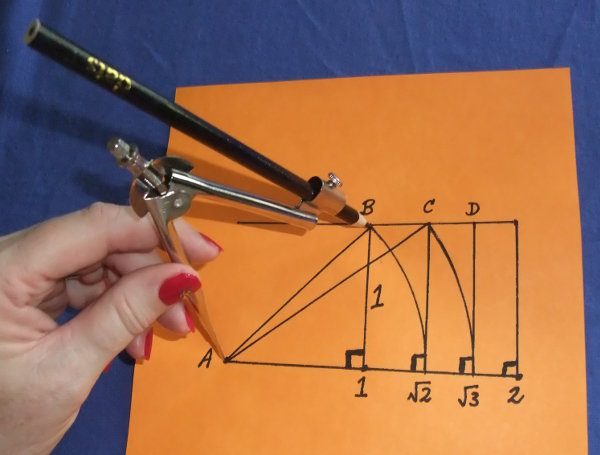# Square Numbers And Square Roots

Square Numbers, also called Perfect Squares, are the product of an integer and itself.

Examples to learn are:

• 1 × 1 = 1
• 2 × 2 = 4
• 3 × 3 = 9
• 4 × 4 = 16
• 5 × 5 = 25
• 6 × 6 = 36
• 7 × 7 = 49
• 8 × 8 = 64
• 9 × 9 = 81
• 10 × 10 = 100
• 11 × 11 = 121
• 12 × 12 = 144

Conversely, examples of Square Roots are:

• The square root of 1 equals both –1 and +1.
1 = ±1
• The square root of 4 equals both –2 and +2.
4 = ±2
• The square root of 100 equals both –10 and +10.
100 = ±10

## Example - Estimate a Square Root

Estimate 70 (the square root of 70). Write the answer to 1 decimal place.

The square root of 70 is more than 8 and less than 9. Let's try some possible values...
8.1 × 8.1 = 65.6 (too low)
8.3 × 8.3 = 68.89 (too low)
8.5 × 8.5 = 72.25 (too high)
8.4 × 8.4 = 70.56 (close!)
To 1 decimal place, the square root of 70 is 8.4

## Maths Fun

Use a calculator to find the square roots of these numbers: 121, 12321, 1234321 and 123454321. What do you notice?

## Square Roots with a CompassThe Ancient Greeks worked out the values of square roots by drawing a series of right triangles where one side of each successive triangle is 1 unit (e.g. 1 cm) long. Draw your own diagram and use a ruler to measure the square roots of 2, 3, 4, 5, and so on. Check these values with a calculator.